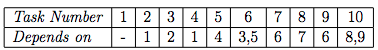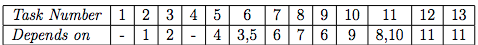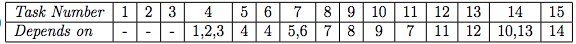# ZIO13004 - Editorial

Editorialist : Sudheera Y S

Problem Link : ZIO 2013 Problem 4

Prerequisites : Graph representation, Permutation, and Combinations

Problem statement :

There are some tasks to be completed and some tasks are dependent on some other tasks i.e we have to do the dependent task before doing the current task ( Note : it is not necessary to do it immediately before ). Find the number of ways to complete all the tasks which satisfies the above condition

Idea :

We will represent this problem as a graph more precisely DAG ( directed acyclic graphs ) and group them and then find the number of permutations and compute the answerRepresenting this as graph :

Let us now do the groups hereIn the above example , we see that 2 is dependent on 3 and 4 is dependent on 5 , but …… can we do 5 without doing 2 ? YES ! So we have to consider that combination also

Can we do 4 without doing 3? YES! We have to consider that also …

Can we do 6 without doing 3? NO

Can we do 6 without doing 5? NO

.

.

.

.

So as we can’t do 6 without 2,3,4,5 … we don’t include 6 in the group as we need to all of them before doing it

So what does a group consist of?

For any task in the group, there is at least one task which is not dependent on it. I hope you understood how groups are made… If you didn’t understand then see the below examples for subtasks, it will be easy as there are graphs and allSo making groups makes finding the number of these combinations easier?YES

How?

Suppose we have 1,2,3,4: and

• 1 is dependent on 2
• 3 is dependent on 4

So now we will find the number of combinations of 1,2,3,4 satisfying the above combination :

1234

1324

1342

3412

3142

3124

So here are all the combinations we have to consider … So making groups help us find all the combinations

In the above example, we have tried all possible 4! I.e 24 test cases

But can we use permutation and combination and do it faster?

1,2 should be in order

3,4 should be in order

Note : Here 1 and 2 need not be consecutive they just have to be in order

So now, we have to choose 2 places for 1 and 2 and put 1 in the first place and 2 in the second place as 1 should be done before doing 2 and in this method, we will calculate all the possible positions for 1 and 2 and this can be done in 4C2 = 6

Now there are 2 places remaining out of 4 and we have 3,4 to fill it and as 3 should always be before 4, we should put 3 in the first place of the remaining 2 places and 4 in the second of the 2 remaining places and this can only be done in 1 way

Therefore, the total number of combinations is 4C2 = 6

So now you would have understood why this grouping works and how the number of combinations in a group is calculated …. So now let us move on and make the groups and find the answerNow suppose we make

So here we make groups as follows :

1. Tasks 1 ( we aren’t allowed to do any task without doing the first task )
2. Tasks 2,3,4,5 ( we can do 5 without doing 2 and we can complete task 3 without doing task 5 but we can’t do any other tasks like task 6 without doing any of these 4 tasks , so these 4 are in one group i.e the permutation doesn’t matter for some of these 4)
3. Tasks 6 ( we can’t do any other tasks without doing the 6th task so only 6 in one group)
4. Tasks 7,9,8 ( To do the 10nth task we need to do 7,8,9 but we can do 9 task without doing 7th or 8th task , so these three are in one group )
5. Task 10 ( To do task 10 , we need to do 8,9 , so task 10 is one group )

Now let us see the number of permutations we can do in each group

Note : we have to maintain the order i.e we can’t do group 5 without doing group 2 so we can’t permute the groups but we can permute the elements in the group )

Number of ways to do group 1 : 1 (1)

AND

Number of ways to do group 2 : 6 ( 4C2 = 6 combinations )

AND

Number of ways to do group 3 : 1 (6)

AND

Number of ways to do group 4 : 3 ( 3C1 = 3 combinations )

AND

Number of ways to do group 5 : 1 ( 10)

Total number of ways to complete all the tasks

Note : Here AND signifies that we have to do multiplication

1 * 6 * 1 * 3 * 1 = 18

So we can complete all the tasks in 18 waysRepresenting it as a graph :

Again as we did earlier, here we are going to make some groups as follows :

1. Tasks 1,2,3,4,5 ( Tasks 1,4 are independent of each other and to do the 6th task we need to complete 3 and 5 but 5 is not dependent on 1,2,3 so we group these 5 tasks)
2. Tasks 6 ( To do tasks 7,9 we need to have completed task 6 so we make this as a group)
3. Tasks 7,8,9,10 ( Tasks 7,9 are dependent on 6 but tasks 9,10 are not dependent on tasks 7,8 and tasks 7,8 are not dependent on tasks 9,10 so we group these 5 )
4. Tasks 11 ( To do tasks 11 we need to have completed task 8,10 and both task 12,13 are dependent on task 11 , so we make 11 as a group )
5. Tasks 12,13 ( Both of these are dependent on task 11 and neither of them are dependent on each other, so we group these two tasks )

Computing the number of ways to do the tasks :

Number of ways to do group 1 : 10 ( 5C3 = 10 combinations)

AND

Number of ways to do group 2 : 1 (6)

AND

Number of ways to do group 3 : 6 ( 4C2 = 6 combinations)

AND

Number of ways to do group 4 : 1 (11)

AND

Number of ways to do group 5 : 2 ( (12)(13) , (13)(12) )

Note : Here AND signifies that we have to do multiplication

Total number of ways to complete all the tasks

101612 = 120

So we can complete all the tasks in 120 waysRepresenting this as graph :

So here we make groups as follows :

1. Tasks 1,2,3 ( None of these three tasks are dependent on any of the other tasks therefore they are in one group)
2. Task 4 (there are no tasks which can be done without having the dependency of 4 so 4 is made a group )
3. Tasks 5,6 (we can do tasks 5,6 by 4 and 5 is not dependent on 6 and 6 is not dependent on 5 so 5,6 are grouped together )
4. Tasks 7 ( task 7 is dependent on 5,6 and we can’t do any other task without doing so 7 is made one group)
5. Tasks 8,9,10,11,12,13 ( tasks 9,10 depend on 8 and tasks 12,13 depend on 11 and 11,12,13 are not depended on 8,9,10 and tasks 8,9,10 are not dependent on tasks 11,12,13 so these 6 tasks are grouped together )
6. Task 14 (to do task 14 we have to complete tasks 10,13 and we cant do any task without 14 so 14 is one group )
7. Task 15 (to do task 15 we have to complete task 14 so 15 is in one group)

Now let us see the number of permutations we can do in each group :

Number of ways to do group 1 : 3! = 6 (123 , 132 , 231 , 213 , 312 , 321)

AND

Number of ways to do group 2 : 1 (4)

AND

Number of ways to do group 3 : 2 (56 , 65)

AND

Number of ways to do group 4 : 1 (7)

AND

Number of ways to do group 5 : 20 ( 6C3 = 20 combinations )

AND

Number of ways to do group 6 : 1 (14)

AND

Number of ways to do group 7: 1 (15)

Note : Here AND signifies that we have to do multiplication

Total number of ways to complete all the tasks

612201*1 = 240

So we can complete all the tasks in 240 ways

Hope you understoodSpecial thanks to @avijit_agarwal for helping me do the (complex) maths and to @g_ajeet_11000 for helping me make the graphs so nice